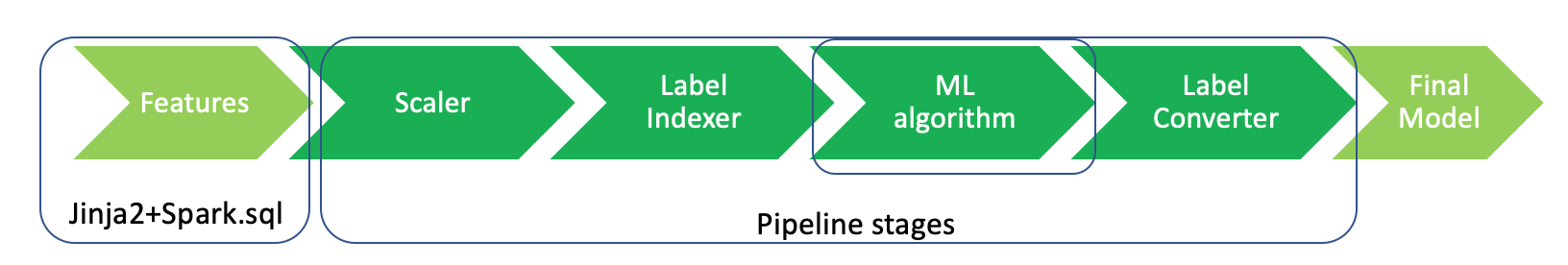# 20. Automation for Cloudera Distribution Hadoop¶

CDH (Cloudera Distribution Hadoop) is the most complete, tested, and widely deployed distribution of Apache Hadoop. A lot of small or middle size companies are using CHD. While Cloudera does not support IPython or Jupyter notebooks on CDH and the Cloudera Data Science Workbench is expensive, many compaies are using `CDH+zeppelin` or `CDH+jupyterhub` infrastructure. This infrastructure works pretty well, but it’s inconvenient for Data Engineer or Data Scientist to do automation during the production process. This chapter will cover how to use Jinja2, spark sql and ML Pipelines to implement the automation for Cloudera Distribution Hadoop.

## 20.1. Automation Pipeline¶

The automation pipeline mainly contains two parts:

1. Jinja2 + spark sql for data clean and manipulation automation
2. ML Pipelines for Machine Leanring automation## 20.2. Data Clean and Manipulation Automation¶

### 20.2.1. Jinja 2¶

Jinja is a modern and designer-friendly templating language for Python, modelled after Django’s templates. Use Jinja2 to generate SQL query will need two steps:

1. Get template
```temp = """
SELECT project, timesheet, hours
FROM timesheet
WHERE user_id = {{ user_id }}
{% if project_id %}
AND project_id = {{ project_id }}
{% endif %}
"""
```
1. render the tempalte
```args = {"user_id": u"runawayhorse",
"project_id": 123}

query=  Template(temp).render(args)

print(query)
```

Then, you will get the following SQL query:

```SELECT project, timesheet, hours
FROM timesheet
WHERE user_id = runawayhorse

AND project_id = 123
```

Note

The Jinja is smart then you think. If you try this

```args = {"user_id": u"runawayhorse"}

query=  Template(temp).render(args)

print(query)
```

Then, you will get the following SQL query:

```SELECT project, timesheet, hours
FROM timesheet
WHERE user_id = runawayhorse
```

If you have a long query, you can use Iinja `get_template` to read the tempalte:

```import os
from jinja2 import Template

path = os.path.abspath(os.path.join(sys.path))
try:
os.mkdir(path)
except OSError:
pass
os.chdir(path)
print(path)

template = jinja_env.get_template('test.sql')
query = template.render(states=states)
print(query)
```

with `test.sql` file is as follows:

```select id
{% for var in states %}
, (CASE WHEN (off_st = '{{var}}') THEN 1 ELSE 0 END)  AS off_st_{{var}}
{% endfor %}
FROM table1
```

Then you will get the following query:

```select id

, (CASE WHEN (off_st = 'MO') THEN 1 ELSE 0 END)  AS off_st_MO

, (CASE WHEN (off_st = 'KS') THEN 1 ELSE 0 END)  AS off_st_KS

, (CASE WHEN (off_st = 'KY') THEN 1 ELSE 0 END)  AS off_st_KY

, (CASE WHEN (off_st = 'OH') THEN 1 ELSE 0 END)  AS off_st_OH

FROM table1
```

### 20.2.2. Spark SQL¶

Spark SQL at here will be called to excute SQL or HiveQL queries which generated by Jinjia2 on existing warehouses.

```# without output
spark.sql(query)

# with output
df = spark.sql(query)
```

## 20.3. ML Pipeline Automation¶

I will not cover the details of the ML Pipeline at here, the interested reader is referred to ML Pipelines . The The main steps for defining the stages are as follows:

```scalering ='Standard'

from pyspark.ml.feature import Normalizer, StandardScaler, MinMaxScaler
if scalering=='Normal':
scaler = Normalizer(inputCol="features", outputCol="scaledFeatures", p=1.0)
elif scalering=='Standard':
scaler = StandardScaler(inputCol="features", outputCol="scaledFeatures",
withStd=True, withMean=False)
else:
scaler = MinMaxScaler(inputCol="features", outputCol="scaledFeatures")

from pyspark.ml.feature import StringIndexer
labelIndexer = StringIndexer(inputCol='label',
outputCol='label').fit(transformed)

from pyspark.ml.feature import IndexToString
# Convert indexed labels back to original labels.
labelConverter = IndexToString(inputCol="prediction", outputCol="predictedLabel",
labels=labelIndexer.labels)

from pyspark.ml.classification import LogisticRegression
ml = LogisticRegression(featuresCol='scaledFeatures', labelCol='label', maxIter=100, regParam=0.01, elasticNetParam=0.6)

# Chain indexers and tree in a Pipeline
pipeline_model = Pipeline(stages=[scaler,labelIndexer,ml,labelConverter])

# Train model.  This also runs the indexers.
model = pipeline_model.fit(trainingData)

# Make predictions.
predictions = model.transform(testData)
```

## 20.4. Save and Load PipelineModel¶

```# save PipelineModel
model.write().overwrite().save(out_path)

from pyspark.ml import PipelineModel

```

## 20.5. Ingest Results Back into Hadoop¶

```df.createOrReplaceTempView("temp_table")

query = '''
create table database_name.prediction_{{dt}} AS
SELECT *
FROM temp_table
'''
output = Template(query).render(dt=dt)
spark.sql(output)
```